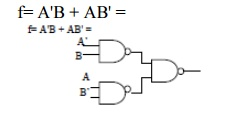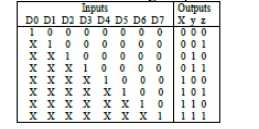Home | | Digital Logic Circuits | Important Short Questions and Answers: Combinational Circuits

# Important Short Questions and Answers: Combinational Circuits

Digital Logic Circuits - Combinational Circuits - Important Short Questions and Answers: Combinational Circuits

COMBINATIONAL CIRCUITS

1. Write the procedural steps for the design of combinational circuits.

Solution

The design of combinational circuit starts from a specification of the problem

culminates in a logic diagram or set of Boolean equations from which the logic diagram can be obtained.

The procedure involves the following steps:

1. From the specifications of the circuit, determine the required number of inputs and outputs, and assign a letter symbol to each.

2. Derive the truth table that defines the required relation ship between inputs and

outputs.

3. Obtain the simplified Boolean functions of each output as function of the input variables.

4. Draw the logic diagram.

5. Verify the correctness of the design.

2. Give the truth table for 4 bit priority encoder.

Solution:

A 4-bit priority encoder (also sometimes called a priority decoder). This circuit basically converts the 4-bit input into a binary representation. If the input n is active, all lower inputs (n-1…0) are ignored:

The circuit operation is simple. Each output is driven by an OR-gate which is connected to the NAND-INV outputs of the corresponding input lines. The NAND gate of each stages receives its input bit, as well as the NAND gate outputs of all higher priority stages. This structure implies that an active input on stage n effectively disables all lower stages n-1.. 0.Note that the circuit function as specified here does not depend at all on the least significant input bit.

A common use of priority encoders is for interrupt controllers, to select the most critical out of multiple interrupt requests. Due to electrical reasons (open collector outputs), priority encoders with active-low inputs are also often used in practice.

3. Draw the Truth Table of Full Adder.

Solution:4. Realize f= A'B + AB' using minimum universal gates.

Solution:5. Draw a tristate inverter and draw its truth table .

Solution:

A tri-state buffer is a useful device that allows us to control when current passes through the device, and when it doesn't. Here are two diagrams of the tri-state buffer.6. Draw a combinational logic circuit which can compare whether two bit binary numbers are same or not.

Solution:Which is similar to EX-NOR operation Y= A’B’+AB

7. What is combinational circuit? Give an example.

Solution:

A combinational circuit consists of logic gates whose outputs at any time are

determined from the present combination of inputs. Examples of combinational circuits are adder, coder, magnitude comparator etc.

8. What are the universal gates?

Solution:

NAND and NOR are universal gates, because they replace all the other gates in a circuit.

9. Obtain 3 level NOR – NOR implementation of f(a,b,c,d,e,f)=[ab+cd ]ef.

f (a, b, c, d, e, f)=[ab+cd ]ef

= abef +cdef

F’= (abef + cdef)’ =(abef)’.(cdef)’

= a+b+e+f . c+d+e+f10.   Draw a 1 to 2-demultiplexer circuit.

Solution:11. Draw a 2 to 1 multiplexer circuit.

Solution12.   What is demux?

Solution:

Demultiplexer is a circuit that receives information on a single line and transmits this information on one of 2n possible output lines. A demultiplexer is a decoder with an enable input.

13. Draw a parity checker circuit for 3 bit binary word x1 x2 x3.

Solution:14.   What does LS in 74LS00 indicate?

Solution:

Low power schottky TTL

15.   Define priority encoder.

Solution:

Encoders establish an input priority to ensure that only the highest-priority input line is encoded. If priority is given to an input with higher subscript number over one with lower subscript number, then if both D2 and D5 are logic-1 simultaneously, the output will be 101 because D5 has a higher priority over D2.Study Material, Lecturing Notes, Assignment, Reference, Wiki description explanation, brief detail
Digital Logic Circuits : Combinational Circuits : Important Short Questions and Answers: Combinational Circuits |